Newton's First Law of Motion and Concept of Inertia, Mass and Momentum

# Newton's First Law of Motion and Concept of Inertia, Mass and Momentum - Science Class 9

 Table of contentsNewton's First Law of MotionInertiaTypes of InertiaInertia and Mass## Newton's First Law of Motion

A body at rest will remain at rest and a body in motion will remain in uniform motion unless an unbalanced forces acts on it to change its state of rest or of uniform motion.
The above law can be understood in two parts.
(i) A body at rest remains at rest unless an external unbalanced force acts on it to change its state of rest.
Ex. Consider a wooden block kept on a horizontal surface at rest. It will remain at rest unless somebody moves it.
(ii) A body in motion will remain in uniform motion unless an external unbalanced force acts on it to change its state of uniform motion.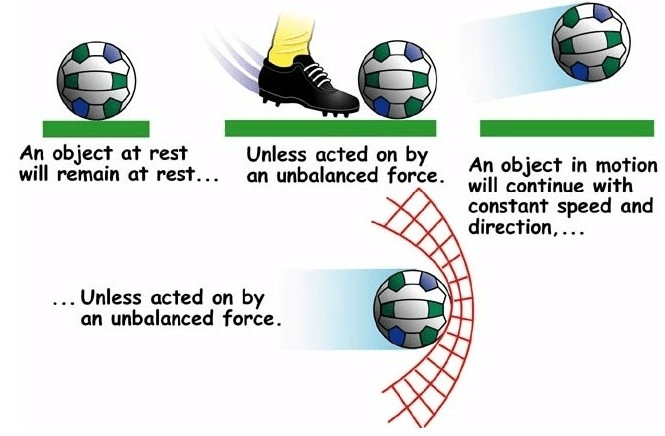Newton's First Law of MotionNewton's first law gives the qualitative definition of force. As it tells us about an agent without which acceleration is not possible.

Newton's first law is also called the law of inertia.

Newton's first law consists of two parts

(i) A body cannot change its state of rest by itself.

(ii) A body cannot change its state of motion by itself.

## Inertia

The tendency of a body to oppose any change in its state of rest or of uniform motion is called inertia of the body.

### Galileo's Law of Inertia

Galileo studied motion of objects on an inclined plane. He found that
(i) objects moving down a smooth inclined plane accelerate.
(ii) objects moving up a smooth inclined plane retard.
(iii) objects moving on a frictionless horizontal plane move with a constant velocity, having neither acceleration nor retardation.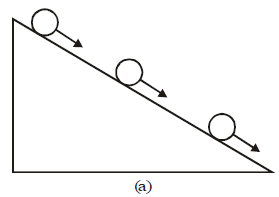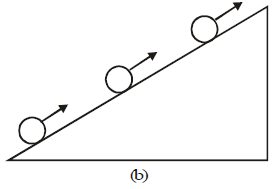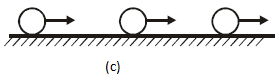In another experiment using a double inclined plane, Galileo observed that
(i) A ball released from rest on one smooth inclined plane rolls down and climbs up the other smooth inclined plane. He found that 'In ideal situation, when there is no friction, the final height of the ball is the same as its initial height. In actual practice, when some friction is there, final height is somewhat less than the initial height. In no case, final height is ever greater than the initial height. When the slopes of the two planes are same, distance covered in rolling down one incline is the same as the distance' covered in climbing up the other incline. This is shown in fig.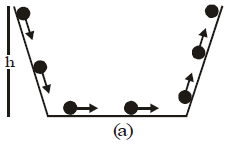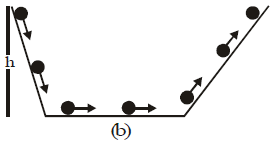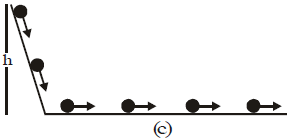(ii) When the slope of second smooth inclined plane is decreased, and the experiment is repeated, the ball still reaches the same final height. But in doing so, it travels a larger distance as shown in fig.
(iii) When the slope of second smooth inclined plane is made zero (i.e., the second plane is made horizontal), the ball travels an infinity distance in the ideal situation (when there is no friction).
From his experiments, Galileo concluded that the state of rest and the state of motion with constant velocity are equivalent. In both cases, no net force is acting on the body.
Galileo emphasized that it is incorrect to assume that a net force is needed to keep a body in uniform motion along a straight line.

Thus, Galileo concluded that if net external force is zero, a body at rest continues to be at rest, and a body in uniform motion continues to move uniformly along the same straight line. This is Galileo's law of inertia.

This, inherent property of all bodies, by virtue of which they cannot change by themselves their state of rest or of uniform motion along a straight line is called Inertia.

## Types of Inertia

(a) Inertia of rest

The resistance offered by a body to change its state of rest is called inertia of rest.
or
The tendency of a body to oppose any change in its state of rest is known as inertia of rest.
Examples:
(i) When a branch of a tree is shaken vigorously, ripe fruits get detached and fall. This is because the branch comes in motion but the fruits at rest tend to remain at rest due to inertia of rest and get detached. After the fruits get detached, gravity plays its role in making the fruits fall.
(ii) For removing dust from a carpet, we hang the carpet and then beat it with a stick. Due to the beating action, the carpet moves along the stick and the dust particle, in a tendency to remain at rest, get detached from the carpet.
(iii) When you hit a striker on a pile of carrom counters, you will observe that only the lowest counter moves away. The rest of the pile remains in the original position.
(iv) A bullet fired from a gun makes a small hole in the window pane while passing through it, but the stone striking the window pane breaks it into pieces. When a bullet strikes the window pane, only a small portion of the window pane where the bullet strikes comes in motion because the bullet makes contact with the pane for a very short time due to its high speed. On the other hand, the remaining portion of the window pane remains at rest due to inertia of rest. Thus, a small hole is made by the bullet in the window pane.

The speed of the stone is very small compared to the speed of the bullet. So the stone makes contact with the window pane for longer period of time. During this longer time. The whole window pane comes in motion and hence breaks into pieces.
(v) Suppose we are standing in a stationary bus and the driver starts the bus suddenly. We get thrown backward with a jerk.
(vi) When a horse starts suddenly, the rider tends to fall backwards on account of inertia of rest of upper part of the body.

Activity
What happens when a quick jerk is given to a smooth thick cardboard placed on a tumbler with a small coin placed on the cardboard ? The coin will fall in the tumbler. Why?

The coin was initially at rest. When the cardboard moves because of the jerk, the coin tends to remain at rest due to inertia of rest. When the cardboard leaves contact with the coin, the coin falls in the tumbler on account of gravity.

(b) Inertia of motion

The resistance offered by a body to change its state of uniform motion is called inertia of motion.
or
The tendency of a body to a oppose any change in its state of uniform motion is known as inertia of motion.

Examples:
(i) An athlete runs for a while before taking a long jump. The motion gained by the athlete helps him to take a longer jump.
(ii) When we switch off a fan, it continues to rotate for a while due to inertia of motion.
(iii) A person jumping out of a moving bus in the direction of motion of bus falls forwards. This is because when his foot touches the ground the foot comes to rest, but the upper part of his body in motion, tends to remain in motion.
(iv) Suppose we are standing in a moving bus, and the driver stops the bus suddenly. We are thrown forward with a jerk. Let us understand why.
As the bus is suddenly stopped, our feet stop due to friction which does not allow relative motion between the feet and the floor of the bus. But the rest of our body continues to move forward due to inertia. That is why we are thrown forward. The restoring muscular forces exerted on our body by our feet come into play and bring the body to rest.
(v) When a horse at full gallop stops suddenly, the rider falls forward on account of inertia of motion.

(c) Inertia of direction
The resistance offered by a body to change its direction of motion is called inertia of direction.
or
The tendency of a body oppose any change in its direction of motion is known as inertia of motion.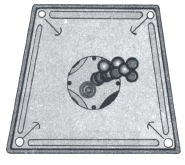Examples:
(i) When a bus suddenly takes a turn, the passengers sitting casually experience a jerk in the outward direction. This happens because the passenger tends to remain in its original direction of motion due to inertia of direction.
(ii) Why are mudguards used over the wheels of a vehicle?' This is because the mud sticking in grooves of the tyre fly off tangentially when the wheel moves at a high speed. To protect the rider from this mud, mudguards are used.
(iii) Suppose a stone tied to a string is whirled around against in a circle. What happens when the string is cut? The stone flies off tangentially due to inertia of direction.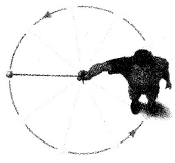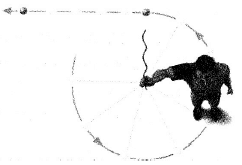(iv) While sharpening the edge of a knife, it is rubbed against a rotating grinding wheel. ,The sparks produced leave the grinding wheel tangentially due to inertia of direction.

## Inertia and Mass

Consider two stationary objects say a small table and a big table. Now if we apply the same amount of force on these two tables, then it is difficult to move the bigger table than the smaller table. It means, the heavy table oppose more to the change in its state of rest than the light or small table. Thus, greater is the mass, greater is the inertia. Hence, inertia of a body is equal to the mass of the body.

The inertia of an object is measured by its mass, a large mass, such as that of a freight train, indicating a large inertia.

Inertia is the natural tendency of an object to remain at rest or in motion at a constant speed along a straight line. The mass of an object is a quantitative measure of inertia.

### Linear Momentum

• Definition: The product of mass and velocity of a body is called Linear momentum, it is denoted by P.
Linear momentum = mass × velocity
• The momentum of the moving body is proportional to
(i) mass of the body, and
(ii) velocity of the body.
• Momentum is a vector quantity.
• Magnitude of momentum, P = mass × speed
or P = mu
• Direction of momentum of a body is same as that of the direction of the velocity of the body.
• Units of Momentum
Momentum = mass × velocity
Unit of momentum = unit of mass × unit of velocity
S.I. unit of momentum is kg ms1.
In CGS system, unit of momentum is g cm1.
The other unit of momentum is N-s.

Note:
(i) Linear momentum has a sense of direction. Therefore it is a vector quantity.
(ii) The direction of linear momentum of a body at any instant is same as that of the velocity of the body at that instant.
(iii) A body moving in a curved path, the direction of velocity at a point is a tangent drawn at that point. Therefore, the direction of linear momentum is also tangential.

The document Newton's First Law of Motion and Concept of Inertia, Mass and Momentum | Science Class 9 is a part of the Class 9 Course Science Class 9.
All you need of Class 9 at this link: Class 9

## Science Class 9

66 videos|355 docs|97 tests

## FAQs on Newton's First Law of Motion and Concept of Inertia, Mass and Momentum - Science Class 9

 1. What is Newton's First Law of Motion?Ans. Newton's First Law of Motion states that an object at rest will stay at rest, and an object in motion will stay in motion with a constant velocity unless acted upon by an external force.
 2. What is inertia?Ans. Inertia is the tendency of an object to resist changes in its state of motion. It is directly related to an object's mass, where objects with greater mass have more inertia.
 3. What are the types of inertia?Ans. There are three types of inertia: 1. Inertia of rest: The tendency of an object to remain at rest when no external force acts upon it. 2. Inertia of motion: The tendency of an object to continue moving with the same velocity in a straight line unless acted upon by an external force. 3. Inertia of direction: The tendency of an object to resist changes in its direction of motion.
 4. How does inertia relate to mass?Ans. Inertia is directly related to an object's mass. The greater the mass of an object, the greater its inertia. This means that objects with larger masses require more force to change their state of motion compared to objects with smaller masses.
 5. What is the relationship between Newton's First Law of Motion and the concept of momentum?Ans. Newton's First Law of Motion is closely related to the concept of momentum. Momentum is the product of an object's mass and velocity and represents the quantity of motion it possesses. According to Newton's First Law, an object in motion will stay in motion with a constant velocity unless acted upon by an external force. This means that the momentum of an object will remain constant unless an external force is applied to change it.

## Science Class 9

66 videos|355 docs|97 testsExplore Courses for Class 9 examSignup to see your scores go up within 7 days! Learn & Practice with 1000+ FREE Notes, Videos & Tests.
10M+ students study on EduRev
Track your progress, build streaks, highlight & save important lessons and more!
Related Searches

,

,

,

,

,

,

,

,

,

,

,

,

,

,

,

,

,

,

,

,

,

,

,

,

;